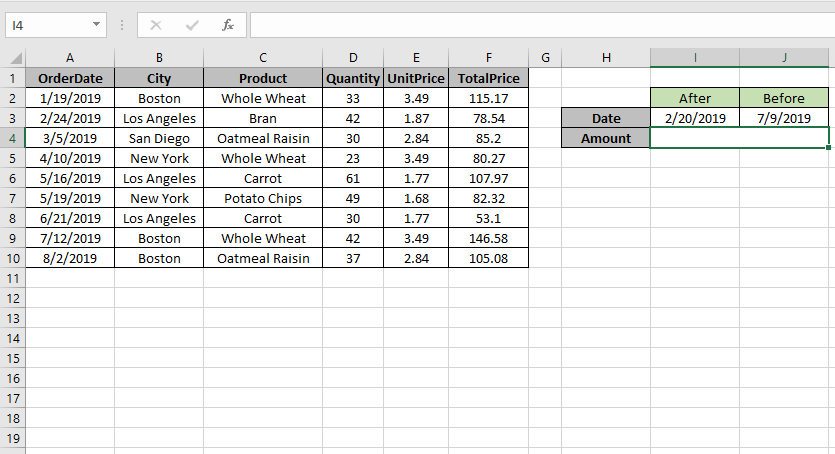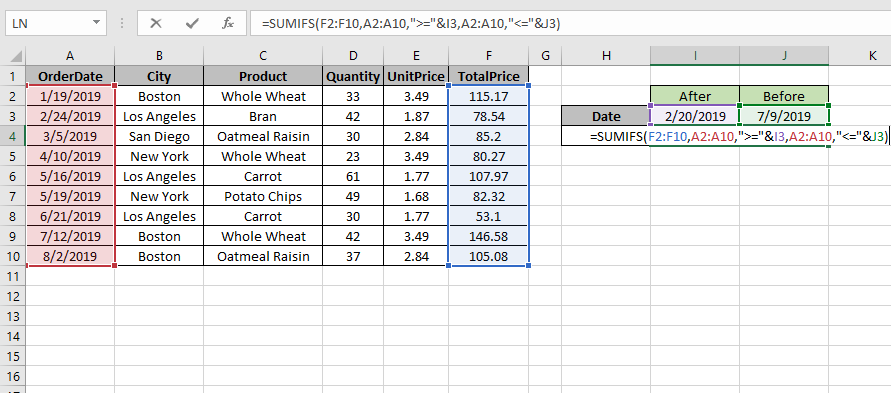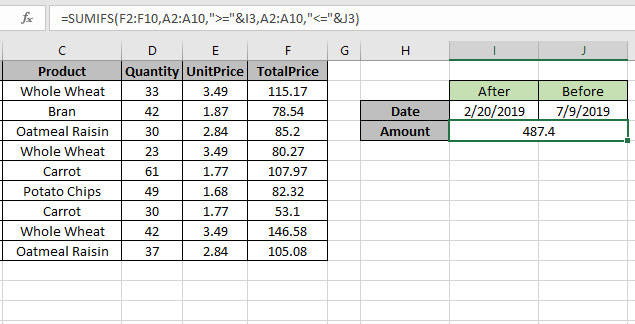# How to get SUM if between dates in Excel

In this article, we will learn about how to Sum values if date is between using SUMIFS in Excel.

In simple words, while working with long data sheet. Sometimes we need to find the sum if only the value which lay between the required dates or say
we need to add values where data date of the corresponding values is between the two required dates.

SUMIFS function returns the sum of range if date is between the
Syntax:

=SUMIFS(Sum_range , range ,">=" & date1, range, "<=" & date2)

Sum_range : range where sum is required
range : Set of dates
& : operator used to concatenate other operator.

Let’s understand this function using it an example.Here we need to find the TotalPrice sum if date is between 2/20/2019 (after) & 7/9/2019 (before).
So we will use the formula to get the amount

=SUMIFS (F2:F10 , A2:A10 , ">=" & I3, A2:A10 , "<=" & J3)

F2:F10 : Sum_range
A2:A10 : range where condition is applied
">=" & I3 : greater than date value in I3(2/20/2019).
"<=" & J3 : less than date value in J3.(7/9/2019).Use the formula as stated above and click Enter.As you can see the formula returns 487.4, the Totalprice between dates.

For customization just change the dates in I3 & J3 cell and get the results with the formula.

Hope you understood how to get the sum if between dates in Excel. Explore more articles on Excel SUMIF function here. Please feel free to state your query or feedback for the above article.

Popular Articles

50 Excel Shortcut to Increase Your Productivity : Get faster at your task. These 50 shortcuts will make you work even faster on Excel.

How to use the VLOOKUP Function in Excel : This is one of the most used and popular functions of excel that is used to lookup value from different ranges and sheets.

How to use the COUNTIF function in Excel : Count values with conditions using this amazing function. You don't need to filter your data to count specific values. Countif function is essential to prepare your dashboard.

How to use the SUMIF Function in Excel : This is another dashboard essential function. This helps you sum up values on specific conditions.

Terms and Conditions of use

The applications/code on this site are distributed as is and without warranties or liability. In no event shall the owner of the copyrights, or the authors of the applications/code be liable for any loss of profit, any problems or any damage resulting from the use or evaluation of the applications/code.